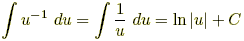# Lecture in Integration of the Powers of the Trigonometric Functions

(Last Updated On: December 21, 2017)

In this topic we are going to learn how to integrate certain combinations of trigonometric functions. We will be using some techniques to help us make it easy for us to evaluate each of them. First let’s start off with the review of the basic formula of Integration by Substitution, I know at this point you could perfectly evaluate integrals using this method.

Formula:, where n ≠ –1, where n = –1

### Integration of the powers of sine and cosine functions

• Case 1. Has the form ∫sinnax cosax dx. I believe this is the basic and the easiest to evaluate because of the presence of the cosine.
• Examples 1: ∫sin53x cos3x dx

Solution: Let u = sin3x, du = 3cos3x dx

=

• Case 2. Has the form ∫cosnax sinax dx. In this case instead sine, the one with the exponent is the cosine and it is a lot easier to evaluate because of the presence of sine.
• Examples 2: ∫cos34x sin4x dx

Solution: Let u = cos4x, du = -4sin4x dx

=

• Case 3. Has the form ∫sinmax cosnax dx where m is odd integer. The first step is to factor sin x, then change the remaining factor into sin2ax = 1 – cos2ax.
• Examples 3:

∫sin33x dx = ∫sin23x ∙ sin3x dx
= ∫(1 – cos23x) sin3x dx
= ∫sin3x dx – ∫cos23x sin3x dx
=

Examples 4:

∫sin52x cos22x dx = ∫sin42x ∙ sin2x cos22x dx
= ∫(sin22x)2 cos22x sin2x dx
= ∫(1 – cos22x)2 cos22x sin2x dx
= ∫(1 – 2cos22x + cos42x) cos22x sin2x dx
= ∫cos22x sin2x dx – 2∫cos42x sin2x dx + ∫cos62x sin2x dx

Let u = cos2x, du = -2sin2x dx

=

• Case 4. Has the form ∫sinmax cosnax dx where n is odd integer. Here we can factor cos x, then change the remaining factor into cos2ax = 1 – sin2ax.
• Examples 5:

∫cos34x dx = ∫cos24x ∙ cos4x dx
= ∫(1 – sin24x) cos4x dx
= ∫cos4x dx – ∫sin24x cos4x dx
=

Examples 6:

sin2x cos3x dx = ∫sin2x cos2x ∙ cosx dx
= ∫sin2x (1 – sin2x) cosx dx
= ∫(sin2x – sin4x) cosx dx

Let u = sinx, du = cosx dx

=

• Case 5. Has the form ∫sinmax cosnax dx where m and n are even integers. In this case we will be using the half-angle identities below.
• and

Examples 7:

sin2x dx = ½∫(1 – cos2x)dx
= ½∫dx – ½∫cos2x dx
=

Examples 8:

sin2x cos2x dx = ∫½(1 – cos2x)½(1 + cos2x) dx
= ¼∫(1 – cos22x) dx
= ¼∫[1 – ½(1 + cos4x)] dx
= ⅛∫(1 – cos4x) dx
=
• Case 6. Has the form ∫sinaxcosbx dx, ∫sinaxsinbx dx, ∫cosaxcosbx dx. To find the integrals for this kind of combination of trigonometric functions, we will be needing the identities below.
• Examples 9:

∫sin6xcos3x dx = ½∫(sin9x + sin3x) dx
= ½∫sin9x dx + ½∫sin3x dx
=

Examples 10:

∫sin5xsin2x dx = ½∫(cos3x – cos7x) dx
= ½∫cos3x dx – ½∫cos7x dx
=

### Integration of the powers of tangent and secant functions

• Case 1. Has the form ∫tanmu secnu du. In this case, when m is odd factor out sec u tan u du and change the remaining tangent into secant using the identity, tan2u = sec2u – 1.
• Examples 11:

∫tan3x sec3x dx = ∫tan2x sec2x ∙ secx tanx dx
= ∫(sec2x – 1)sec2x ∙ secx tanx dx
= ∫(sec4x – sec2x)secx tanx dx

Let u = secx, du = secx tanx dx

= ∫ u4 du – u2 du
=

• Case 2. Has the form ∫tanmu secnu du. When n is even greater than 2, factor out sec2u du and replace the remaining secant by tangent using the identity, sec2u = 1 + tan2u.
• Examples 12:

∫tan5x sec4x dx = ∫tan5x sec2x ∙ sec2x dx
= ∫tan5x(1 + tan2x)sec2x dx
= ∫tan5x sec2x dx + ∫tan7x sec2x dx

Let u = tanx, du = sec2x dx

= ∫ u5 du + u7 du
=

• Case 3. Has the form ∫tanmu secnu du. When m is even the integrand is tangent only use the identity, tan2u = sec2u – 1.
• Examples 13:

∫tan4x dx = ∫tan2x ∙(sec2x – 1) dx
= ∫tan2x sec2x dx – ∫tan2x dx
= ∫tan2x sec2x dx – ∫(sec2x – 1) dx

Let u = tanx, du = sec2x dx

= ∫u2 du – ∫du + ∫dx
=

### Integration of the powers of cotangent and Cosecant functions

• Case 1. Has the form ∫cotmu cscnu du. When m is odd, factor out csc u cot u du and change the remaining cotangent into cosecant using the identity, cot2u = csc2u – 1.
• Examples 14:

∫cot32x csc32x dx = ∫cot22x csc22x ∙ (cot2x csc2x dx)
= ∫(csc22x – 1)csc22x ∙ cot2x csc2x dx
= ∫(csc42x – csc22x)cot2x csc2x dx

Let u = csc2x, du = -2csc2x cot2x dx

= -½∫u4 du + ½∫u2 du
=

• Case 2. Has the form ∫cotmu cscnu du. When n is even greater than 2, factor out csc2u du and replace the remaining cosecant by cotangent using the identity, csc2u = 1 + cot2u.
• Examples 15:

∫cot4x csc4x dx = ∫cot4x csc2x ∙ csc2x dx
= ∫cot4x(1 + cot2x) csc2x dx
= ∫(cot4x + cot6x) csc2x dx

Let u = cotx, du = -csc2x dx

= -∫u4 du – ∫u6 du
=

P inoyBIX educates thousands of reviewers and students a day in preparation for their board examinations. Also provides professionals with materials for their lectures and practice exams. Help me go forward with the same spirit.

“Will you subscribe today via YOUTUBE?”

Subscribe

PinoyBIX Engineering. © 2014-2020 All Rights Reserved | How to Donate? |